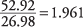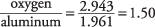## Stoichiometry

The atomic ratios in each compound are also the relative number of atomic mass units of its elements. The first example is nitrous oxide (N 2O), as shown in Table 1.The relative masses were obtained by multiplying the atomic ratios and atomic masses. You can see that a sample of N 2O weighing 44.02 grams contains 28.02 g of nitrogen and 16.00 g of oxygen. The mass percent of each element is calculated from its relative mass divided by the sum of the relative masses. Chemical compounds with integral atomic ratios, like nitrous oxide, are described as stoichiometric compounds, and they permit many simple calculations.

The common oxide of aluminum provides a second example, but this time, begin with the mass percent and deduce the atomic ratio. Careful laboratory analysis of aluminum oxide determines it to be approximately 53 percent aluminum and 47 percent oxygen by mass, as shown in the second column in Table 2.The relative masses are obtained by dividing the mass percentages by the atomic masses from the periodic table.

aluminumoxygenSo, the ratio of oxygen to aluminum in relative atomic masses isIf the aluminum oxide is a stoichiometric compound with whole‐number ratios of the constituent elements, the preceding quotient must be translated into a ratio of integers:This ratio of integers implies that the formula for aluminum oxide is A1 2O 3. In simple compounds, the metallic elements are written before the nonmetals.

Most chemical compounds are stoichiometric, and you should be able to utilize the atomic masses and perform the following calculations:

• Mass percents from the chemical formula
• Chemical formula from the weight percents

These calculations are so basic to the field that you should go back and carefully review the two examples in this section: the calculation of mass percents in N 2O and the inference of the formula for aluminum oxide. Then you can practice stoichiometric calculations on the following pair of problems, which are answered and explained in the

appendix. Many such practice exercises are included in this book so that you can determine whether you understand the major concepts of chemistry. It is well worth your time to study these examples and their explanations in the appendix until you can do the calculations correctly.

• Determine the mass percentages of the three elements in ammonium chloride, which has the formula NH 4C1.
• Infer the simplest chemical formula for potassium copper (II) fluoride, which has this analysis by mass: